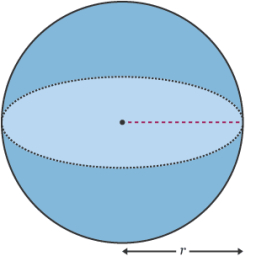# Sphere volume formula

If V=4/3 π r3, find the value of V when r = 7, the value of r when V=113 1/7

V1 =  1436.755
r2 =  3

### Step-by-step explanation:Did you find an error or inaccuracy? Feel free to write us. Thank you!

Emily
what is the shape that you can add on here

Will
i am having trouble with geometry

Tips for related online calculators